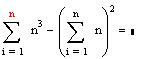Mathematical Software Review (in Progress)

Mathematica 4, Maple 6, Mathcad 2000, NuCalc, GrafEQ13 + 23 + 33 + ... + n3 = (1 + 2 + 3 + ... + n)2 in Mathematica, Maple, and Mathcad

I now have all of the above programs (\$\$\$!), and have started a comparison review.  It's a bit like comparing Euler, Euclid, and Einstein.  Each of the above links will take you to the corresponding home page for these programs.  As a starter, I graphed sin(x sin(y)) = cos(y cos(x)).  The results are beautiful.  Mathematica's graphMaple's graph. Nucalc's graphGrafEQ's graph.  Can Mathcad do this?  I will have much more on these programs in the months to come.  If you know of other mathematical software programs, please contact me.